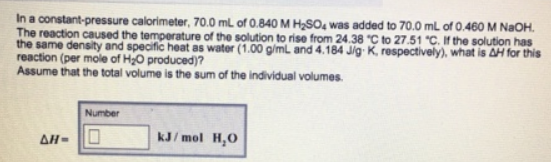# Problem: In a constant-pressure calorimeter, 70.0 mL of 0.840 M H2S04 was added to 70.0 mL of 0.460 M NaOH. The reaction caused the temperature of the solution to nse for 24.38°C - to 27.51°C. If the solution has the same density and specific heat as water (1.00 g/mL and 4.184 J/g • K, respectively), what is ΔH for this reaction (per mole of H2O produced)? Assume that the total volume is the sum of the individual volumes.

###### FREE Expert Solution
87% (446 ratings)###### Problem Details

In a constant-pressure calorimeter, 70.0 mL of 0.840 M H2S04 was added to 70.0 mL of 0.460 M NaOH. The reaction caused the temperature of the solution to nse for 24.38°C - to 27.51°C. If the solution has the same density and specific heat as water (1.00 g/mL and 4.184 J/g • K, respectively), what is ΔH for this reaction (per mole of H2O produced)? Assume that the total volume is the sum of the individual volumes.